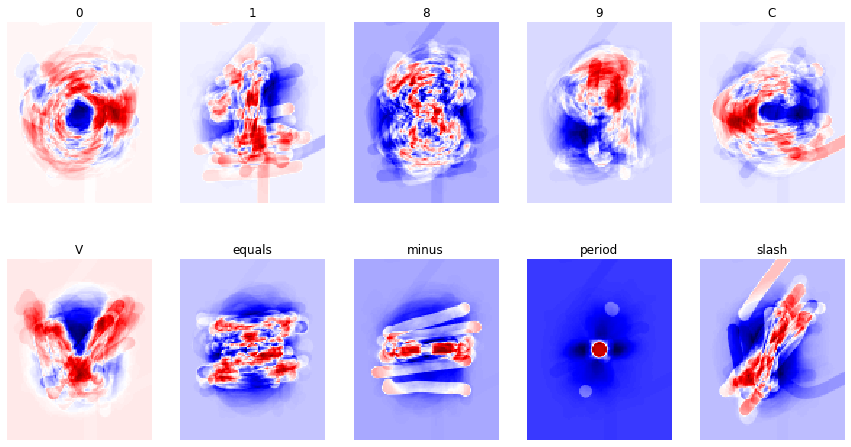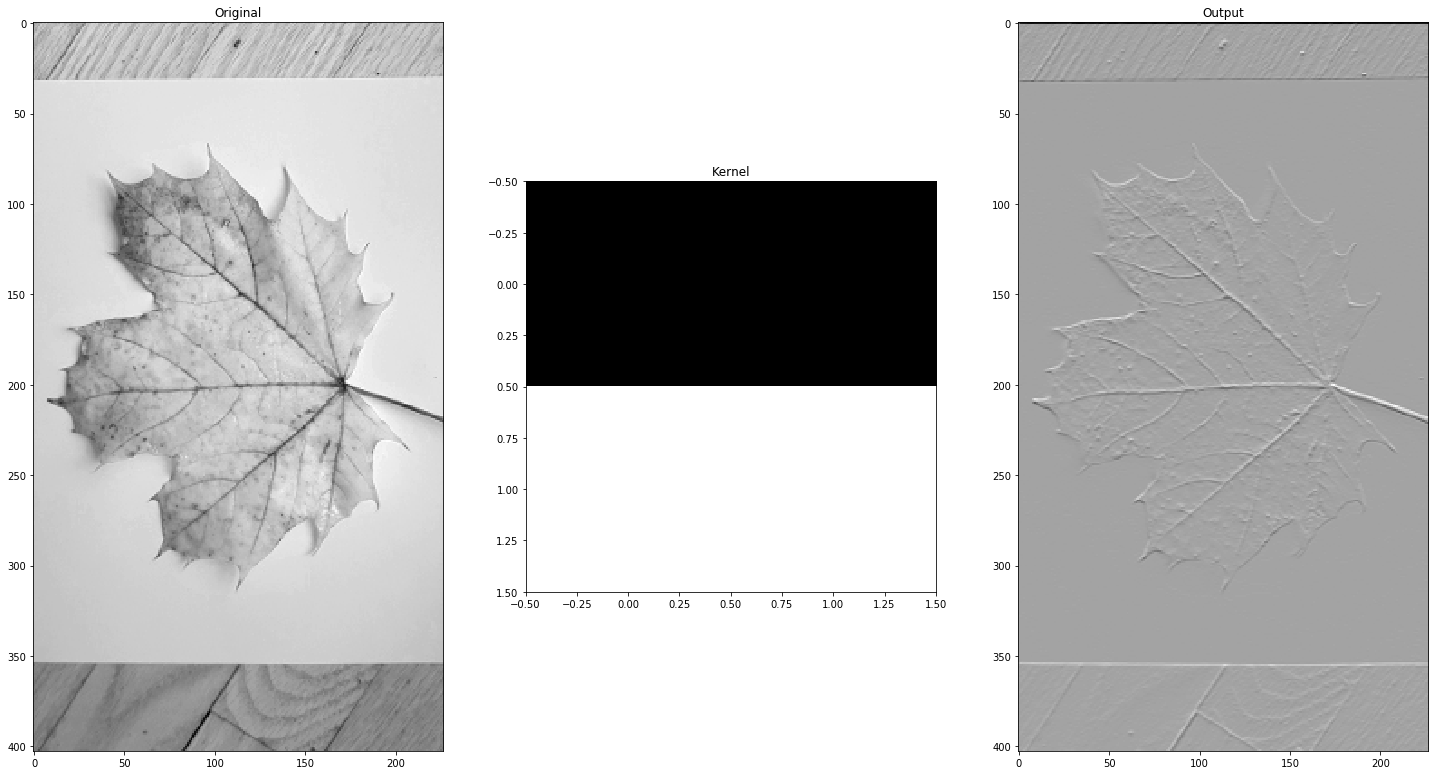In :
import numpy as np
from tensorflow.keras.models import Sequential
from tensorflow.keras.layers import Dense

# build the network
model = Sequential()
from tensorflow.keras.optimizers import SGD
sgd = SGD(learning_rate=0.01, momentum=0.0)#, nesterov=False)
model.compile( optimizer=sgd, loss='categorical_crossentropy', metrics=['accuracy'] )
model.set_weights([np.zeros((12500, 10)),np.zeros((10))])

from PIL import Image
from glob import glob
import matplotlib.pyplot as plt
#%config InlineBackend.figure_format = 'retina' # linux only
#%matplotlib inline
import numpy as np

def charclass(png):     # extract character class name from file name
return png.split('__')[:-4]

from os.path import join
def get_images_and_labels( folder ):
pngs = sorted( glob(join(folder,'*.png')) )
h,w,_ = np.array(Image.open(pngs)).shape
h,w
classes = sorted({charclass(png) for png in pngs}) # ['8','9','minus'] #
n = len(pngs)
c = len(classes)
# Load flattened images as columns of big array X
X = np.empty((h*w,len(pngs)))
for i,png in enumerate(pngs):
X[:,i] = 255 - np.array(Image.open(png))[:,:,0].reshape(h*w)
X /= 255
# Get the true class numbers of all the images
y = [classes.index(charclass(png)) for png in pngs]  # true classes of images
Y = np.zeros((n,c),dtype=int)
Y[ np.arange(n), y ] = 1  # one-hot encoding of true classes
return X.T, Y  # tensorflow wants X the other way

# train the network
model.fit( *get_images_and_labels('pngs'), epochs=20, batch_size=16)

test_loss, test_acc = model.evaluate(*get_images_and_labels('testpngs'), verbose=2)
print('\nTest accuracy:', test_acc)
# get the weights
W,_ = model.get_weights()
#for item in weights:
#    print(item.shape)
WT = W.T

Train on 1514 samples
Epoch 1/20
1514/1514 [==============================] - 1s 822us/sample - loss: 0.8433 - accuracy: 0.8263
Epoch 2/20
1514/1514 [==============================] - 1s 578us/sample - loss: 0.3773 - accuracy: 0.9306
Epoch 3/20
1514/1514 [==============================] - 1s 624us/sample - loss: 0.2921 - accuracy: 0.9538
Epoch 4/20
1514/1514 [==============================] - 1s 673us/sample - loss: 0.2485 - accuracy: 0.9610
Epoch 5/20
1514/1514 [==============================] - 1s 668us/sample - loss: 0.2218 - accuracy: 0.9617
Epoch 6/20
1514/1514 [==============================] - 1s 889us/sample - loss: 0.2022 - accuracy: 0.9643
Epoch 7/20
1514/1514 [==============================] - 1s 635us/sample - loss: 0.1874 - accuracy: 0.9657
Epoch 8/20
1514/1514 [==============================] - 1s 717us/sample - loss: 0.1740 - accuracy: 0.9696
Epoch 9/20
1514/1514 [==============================] - 1s 738us/sample - loss: 0.1636 - accuracy: 0.9696
Epoch 10/20
1514/1514 [==============================] - 1s 646us/sample - loss: 0.1554 - accuracy: 0.9723
Epoch 11/20
1514/1514 [==============================] - 1s 590us/sample - loss: 0.1478 - accuracy: 0.9749
Epoch 12/20
1514/1514 [==============================] - 1s 792us/sample - loss: 0.1411 - accuracy: 0.9729
Epoch 13/20
1514/1514 [==============================] - 1s 593us/sample - loss: 0.1342 - accuracy: 0.9782
Epoch 14/20
1514/1514 [==============================] - 1s 616us/sample - loss: 0.1288 - accuracy: 0.9789
Epoch 15/20
1514/1514 [==============================] - 1s 619us/sample - loss: 0.1238 - accuracy: 0.9808
Epoch 16/20
1514/1514 [==============================] - 1s 632us/sample - loss: 0.1197 - accuracy: 0.9802
Epoch 17/20
1514/1514 [==============================] - 1s 676us/sample - loss: 0.1152 - accuracy: 0.9795
Epoch 18/20
1514/1514 [==============================] - 1s 846us/sample - loss: 0.1116 - accuracy: 0.9835
Epoch 19/20
1514/1514 [==============================] - 1s 877us/sample - loss: 0.1080 - accuracy: 0.9835
Epoch 20/20
1514/1514 [==============================] - 1s 800us/sample - loss: 0.1045 - accuracy: 0.9841
179/1 - 0s - loss: 0.2649 - accuracy: 0.9274

Test accuracy: 0.9273743

In :
# make pictures of the current weights
c = WT.shape
h,w = 125,100
selection = ['0', '1', '8', '9', 'C', 'V', 'equals', 'minus', 'period', 'slash']
plt.figure(figsize=(15,8))
for j in range(c):
plt.subplot(2,c/2,j+1)
plt.imshow( WT[j].reshape(h,w), cmap='seismic' )
plt.axis('off')
plt.title(selection[j])In :


Train on 1514 samples
Epoch 1/2
1514/1514 [==============================] - 1s 818us/sample - loss: 0.8404 - accuracy: 0.8283
Epoch 2/2
1514/1514 [==============================] - 1s 692us/sample - loss: 0.3757 - accuracy: 0.9306
179/1 - 0s - loss: 0.5040 - accuracy: 0.8603

Test accuracy: 0.8603352
(12500, 10)
(10,)

Out:
"\n\n# make pictures of the current weights\nc = WT.shape\nh,w = 125,100\nselection = ['0', '1', '8', '9', 'C', 'V', 'equals', 'minus', 'period', 'slash']\nplt.figure(figsize=(15,8))\nfor j in range(c):\n    plt.subplot(2,c/2,j+1)\n    plt.imshow( WT[j].reshape(h,w), cmap='seismic' )\n    plt.axis('off')\n    plt.title(selection[j])\n"
In :
from scipy import signal
from scipy import misc
from time import time
from PIL import Image
face = np.array(Image.open('maple_leaf_small.jpg'))[:,:,0]
face = np.uint8((255.0*face)/face.max())
kernel = np.outer(signal.gaussian(10, 3), signal.gaussian(10, 3))
kernel = np.array([[-1,-1],[1,1]])
tic = time()
blurred = signal.fftconvolve(face, kernel, mode='same')
toc = time()
print('On image of size',face.shape,', fftconvolve took', toc-tic, 'seconds')
fig, (ax_orig, ax_kernel, ax_blurred) = plt.subplots(1,3,figsize=(25, 25),facecolor='w')
ax_orig.imshow(face, cmap='gray')
ax_orig.set_title('Original')
#ax_orig.set_axis_off()
ax_kernel.imshow(kernel, cmap='gray')
ax_kernel.set_title('Kernel')
#ax_kernel.set_axis_off()
ax_blurred.imshow(blurred, cmap='gray')
ax_blurred.set_title('Output');

On image of size (403, 227) , fftconvolve took 0.006183624267578125 secondsIn :
run draw_one_eye.py

In [ ]:
# Adapted from Adrian Rosebrock https://www.pyimagesearch.com/2019/01/28/keras-regression-and-cnns/

# import the necessary packages
from tensorflow.keras.models import Sequential
from tensorflow.keras.layers import BatchNormalization
from tensorflow.keras.layers import Conv2D
from tensorflow.keras.layers import MaxPooling2D
from tensorflow.keras.layers import Activation
from tensorflow.keras.layers import Dropout
from tensorflow.keras.layers import Dense
from tensorflow.keras.layers import Flatten
from tensorflow.keras.layers import Input
from tensorflow.keras.models import Model

# build the network

def create_cnn(width, height, depth, nfilters=(16, 32, 64), regress=False):
# initialize the input shape and channel dimension, assuming
# TensorFlow/channels-last ordering
inputShape = (height, width, depth)
chanDim = -1

# define the model input
inputs = Input(shape=inputShape)

# loop over the number of filters
for (i, nf) in enumerate(nfilters):
# if this is the first CONV layer then set the input
# appropriately
if i == 0:
x = inputs

# CONV => RELU => BN => POOL
x = Conv2D(nf, (3, 3), padding="same")(x)
x = Activation("relu")(x)
x = BatchNormalization(axis=chanDim)(x)
x = MaxPooling2D(pool_size=(2, 2))(x)

# flatten the volume, then FC => RELU => BN => DROPOUT
x = Flatten()(x)
x = Dense(16)(x)
x = Activation("relu")(x)
x = BatchNormalization(axis=chanDim)(x)
x = Dropout(0.5)(x)

# apply another FC layer: JR not sure what the role of this is
x = Dense(4)(x)
x = Activation("relu")(x)

x = Dense(2, activation="linear")(x)    ################  2D output

# construct the CNN
model = Model(inputs, x)

# return the CNN
return model

import glob
import cv2

# return np.array of images (cv2.imread), slicing off opacity layer if needed
# optionally resize images with cv2.resize
pass

import pandas as pd
# return dataframe of attributes we want to predic
pass

# now actually do it

from sklearn.model_selection import train_test_split
import numpy as np
import os
import matplotlib.pyplot as plt

datafolder = 'one_eye'
datafile   = 'one_eye.txt'
# construct the path to the input .txt file that contains information
# on each image in the dataset and then load the dataset
inputPath = os.path.sep.join([datafolder, datafile])

# load the images and then scale the pixel intensities to the
# range [0, 1]
images = images / 255.0

# partition the data into training and testing splits using 75% of
# the data for training and the remaining 25% for testing
split = train_test_split(df, images, test_size=0.25, random_state=42)   # can alternatively make your own function to do this
(trainAttrX, testAttrX, trainImagesX, testImagesX) = split

# scale and shift the data into [0,1]
trainY = trainAttrX[['x','y']].values / np.array([40,20]) + 0.5	# map into [0,1]  ##### ad hoc here, needs to be modified!
testY  =  testAttrX[['x','y']].values / np.array([40,20]) + 0.5	# map into [0,1]  ##### ad hoc here, needs to be modified!

# create our Convolutional Neural Network and then compile the model
# using mean squared error as our loss

model = create_cnn(90,48, 3, regress=True)
opt = Adam(lr=1e-3, decay=1e-3 / 200)  # more sophisticated than plain gradient descent
model.compile(loss="mean_squared_error", optimizer=opt)

# train the model
print("Training model...")
model.fit(trainImagesX, trainY, validation_data=(testImagesX, testY),
epochs=10, batch_size=8)

model.save('model.h5')  # in case we want to take up training from were we left off

# make predictions on the testing data
print("Predicting ...")
preds = model.predict(testImagesX)
print(preds)
print('Actual')
print(testY)